# Set heatmap cell width in circos.heatmap function

In a previous post I introduced making circular heatmaps with the circlize package. From version 0.4.12, I add a new argument cell_width in circos.heatmap() function so that the heatmap cell width is adjustable.

First I generate a random dataset for making the heatmaps.

library(circlize)
set.seed(123)
mat1 = rbind(cbind(matrix(rnorm(50*5, mean = 1), nr = 50),
matrix(rnorm(50*5, mean = -1), nr = 50)),
cbind(matrix(rnorm(50*5, mean = -1), nr = 50),
matrix(rnorm(50*5, mean = 1), nr = 50))
)
rownames(mat1) = paste0("R", 1:100)
colnames(mat1) = paste0("C", 1:10)
mat1 = mat1[sample(100, 100), ] # randomly permute rows
split = sample(letters[1:5], 100, replace = TRUE)
split = factor(split, levels = letters[1:5])
col_fun1 = colorRamp2(c(-2, 0, 2), c("blue", "white", "red"))

The value for cell_width is simply a numeric vector with the same length as the number of rows of the input matrix. The values in cell_width will be scaled in the function. In the following example, I repetitively set the heatmap cell width with 1, 4, 1, 4, ....

circos.heatmap(mat1, split = split, col = col_fun1, cell_width = rep(c(1, 4), 50))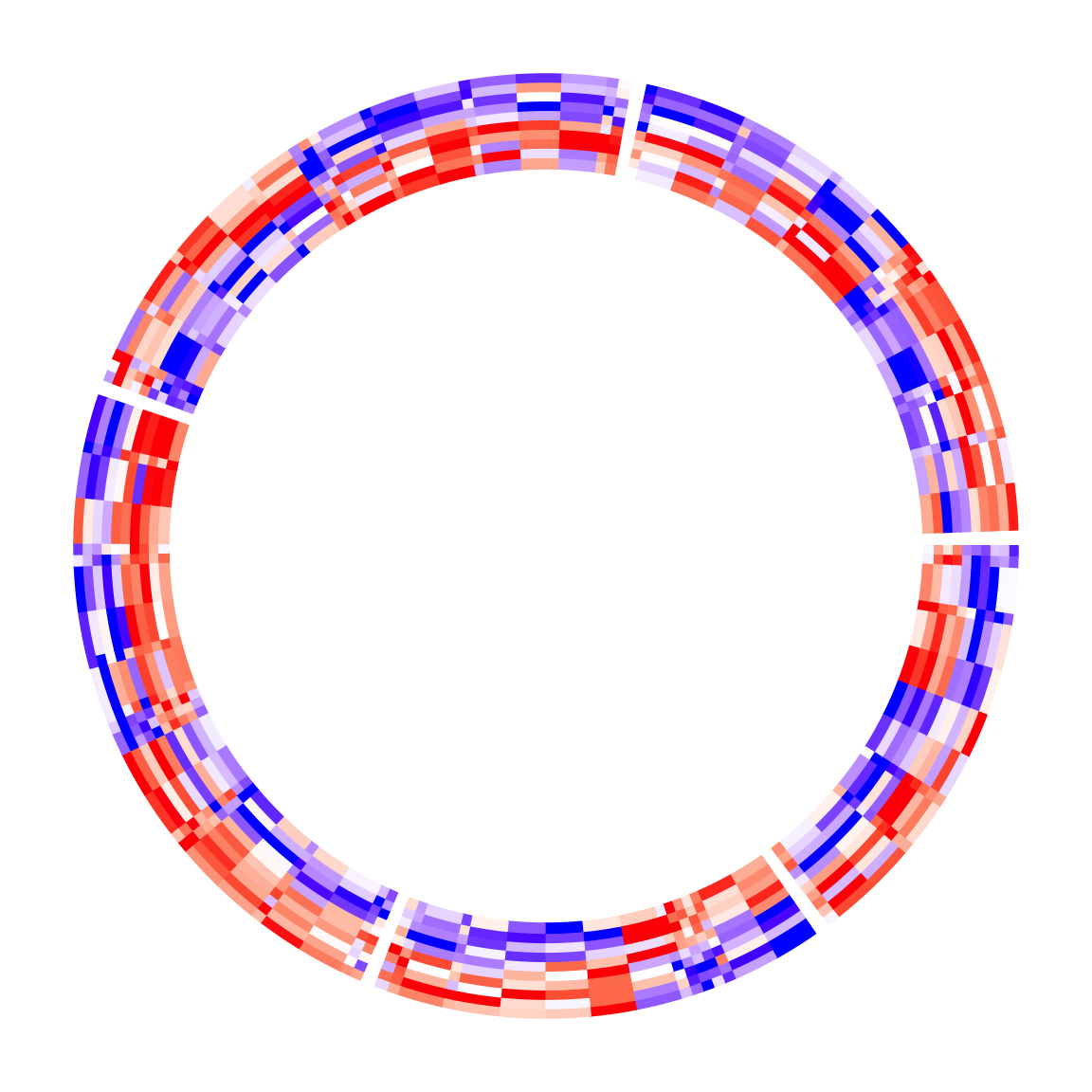circos.clear()

Please note, the order of cell_width should correspond to the original row order of the input matrix, so if clustering or other reordering is applied, the visual effect on heatmap cell widths might be altered.

Since now the heatmap cells do not located evenly, the positions of leaves of the dendrograms are also automatically adjusted:

circos.heatmap(mat1, split = split, col = col_fun1, dend.side = "inside",
cell_width = rep(c(1, 4), 50))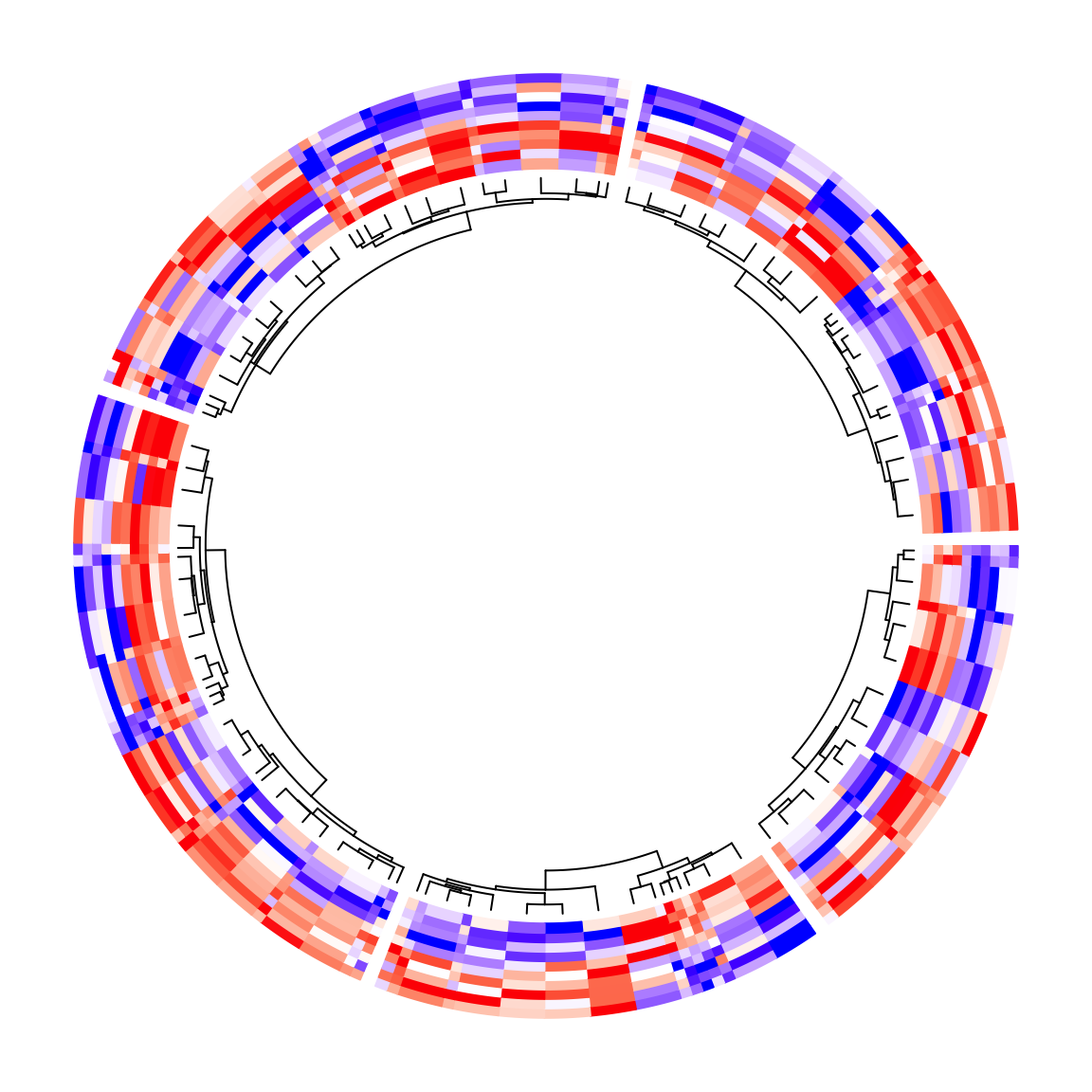circos.clear()

The same for the row names of the matrix. Their positions are adjusted as well.

circos.heatmap(mat1, split = split, col = col_fun1, rownames.side = "inside",
cell_width = rep(c(1, 4), 50))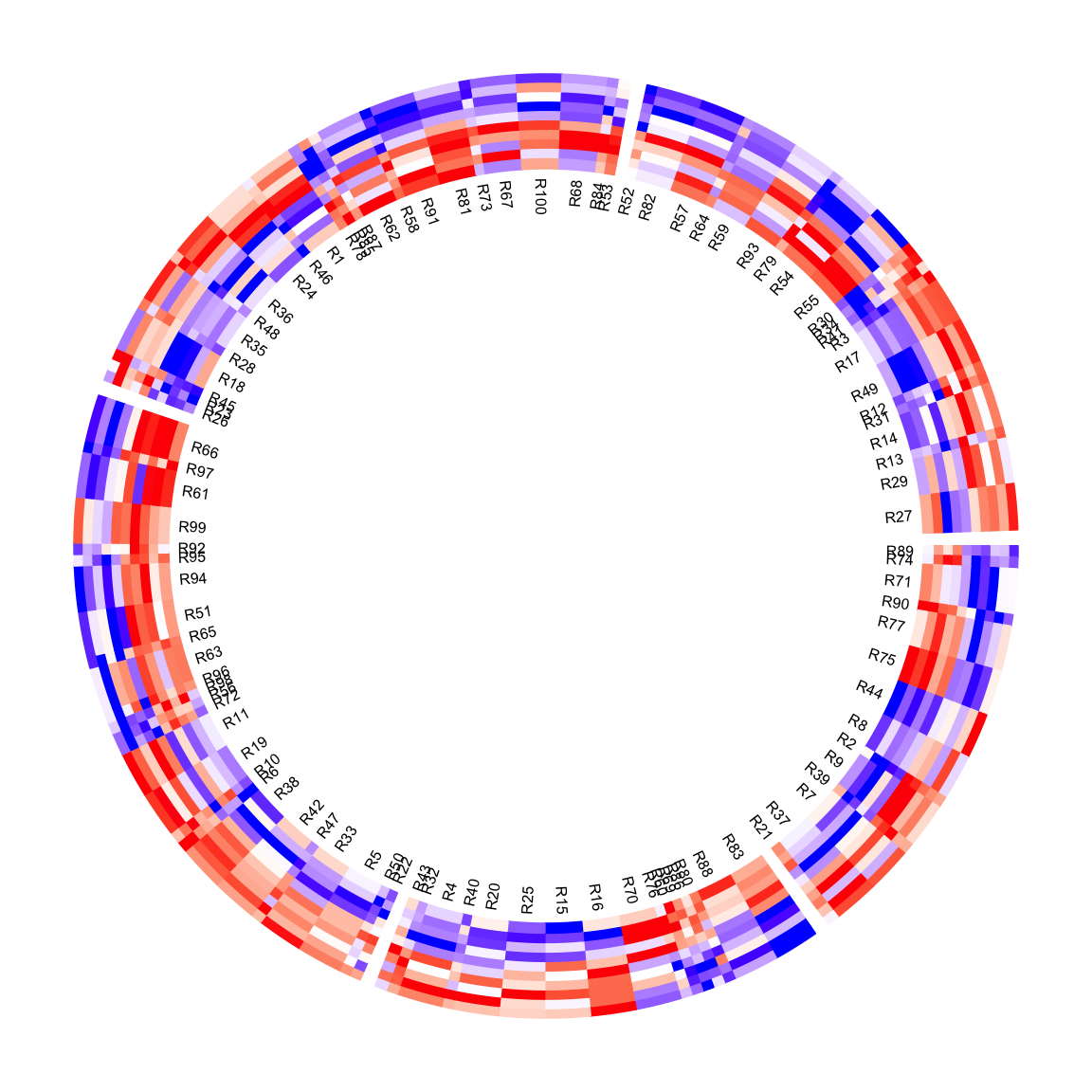circos.clear()

As you have seen, since the heatmap cells have different widths, some labels for small cells overlap. Here we can use circos.labels() (available from version 0.4.12) to add a label track where the positions of labels are automatically adjusted if they overlap. circos.labels() is a general function and it has special settings for circular heatmaps.

circos.heatmap(mat1, split = split, col = col_fun1, cell_width = rep(c(1, 4), 50))
circos.labels(labels = rownames(mat1), cex = 0.5)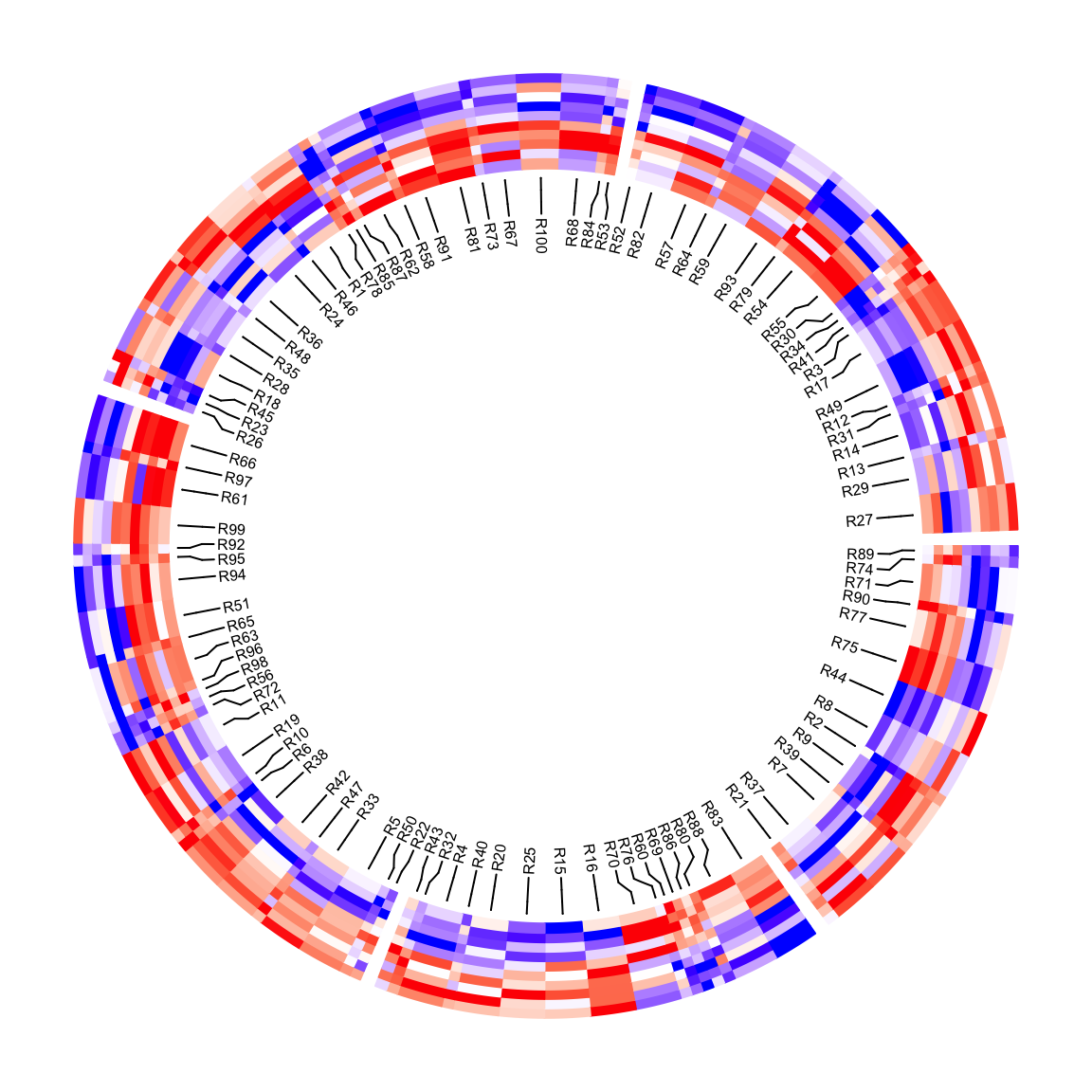circos.clear()

If there are multiple heatmap tracks, cell_width should only be set in the first circos.heatmap() call, then all the remaining heatmap tracks inherit that setting. More generally, cell_width can be set in circos.heatmap.initialize() to control it globally.

mat2 = mat1[sample(100, 100), ] # randomly permute mat1 by rows
col_fun2 = colorRamp2(c(-2, 0, 2), c("green", "white", "red"))

circos.heatmap(mat1, split = split, col = col_fun1, cell_width = rep(c(1, 4), 50))
circos.heatmap(mat2, col = col_fun2)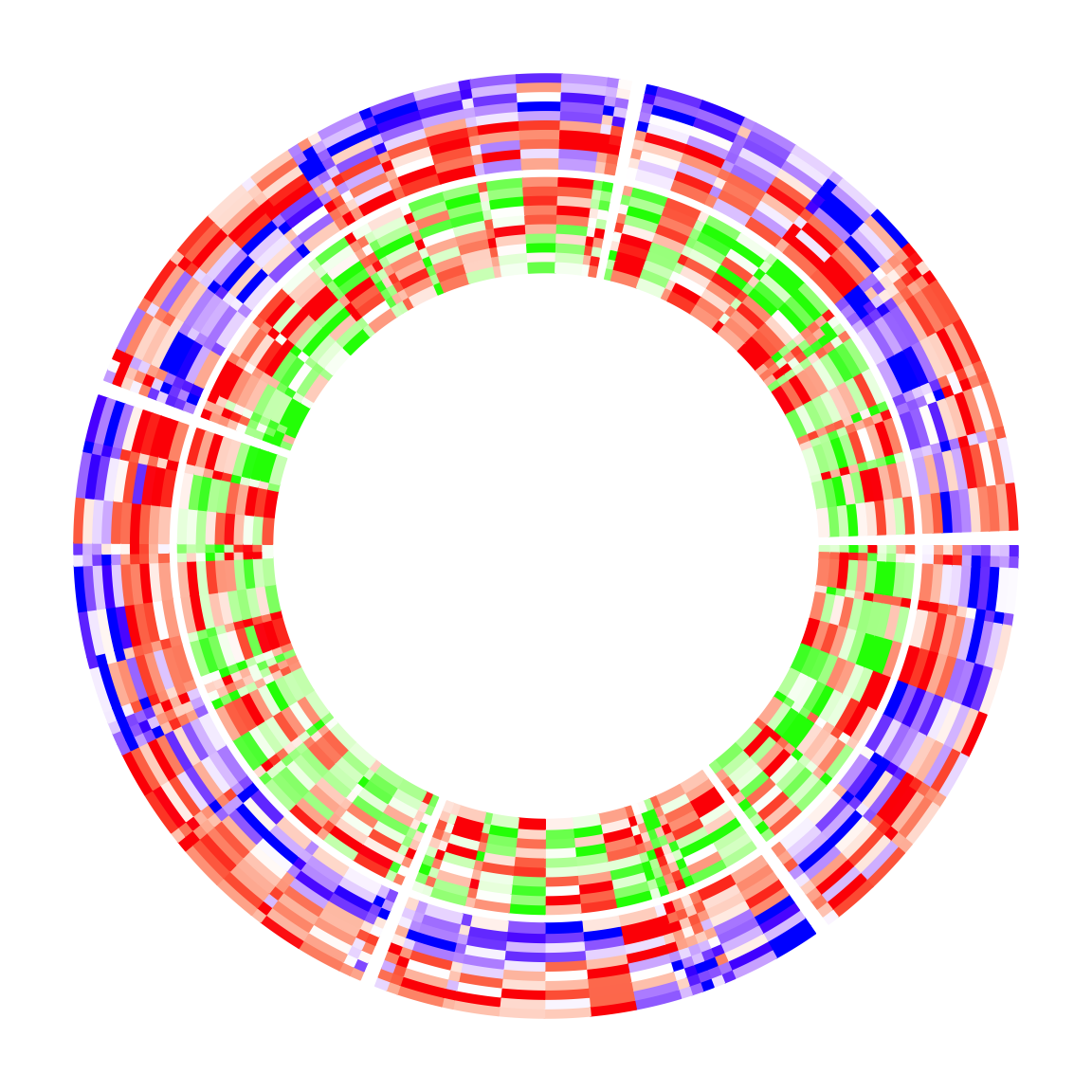circos.clear()

When cell_width is set, the heatmap cells no longer locate at 0.5, 1.5, .... The positions of the heatmap cells as well as their widths can be retrieved with CELL_META$cell_middle and CELL_META$cell_width. In the following example, a track of boxplots and a track of barplots are added where each box and bar are aligned to every heatmap cell. Please note the orders of CELL_META$cell_middle and CELL_META$cell_width correspond to the order of the original matrix.

circos.heatmap(mat1, split = split, col = col_fun1, cell_width = rep(c(1, 4), 50))
circos.track(ylim = range(mat1), panel.fun = function(x, y) {
m = mat1[CELL_META$subset, 1:5, drop = FALSE] n = nrow(m) circos.boxplot(t(m), pos = CELL_META$cell_middle,
box_width = CELL_META$cell_width*0.8, pch = 16, cex = 0.3) circos.lines(CELL_META$cell.xlim, c(0, 0), lty = 2, col = "grey")
}, cell.padding = c(0.02, 0, 0.02, 0))

circos.track(ylim = range(mat1[, 1]), panel.fun = function(x, y) {
x = mat1[CELL_META$subset, 1] circos.barplot(x, pos = CELL_META$cell_middle,
bar_width = CELL_META\$cell_width*0.8)
}, cell.padding = c(0.02, 0, 0.02, 0))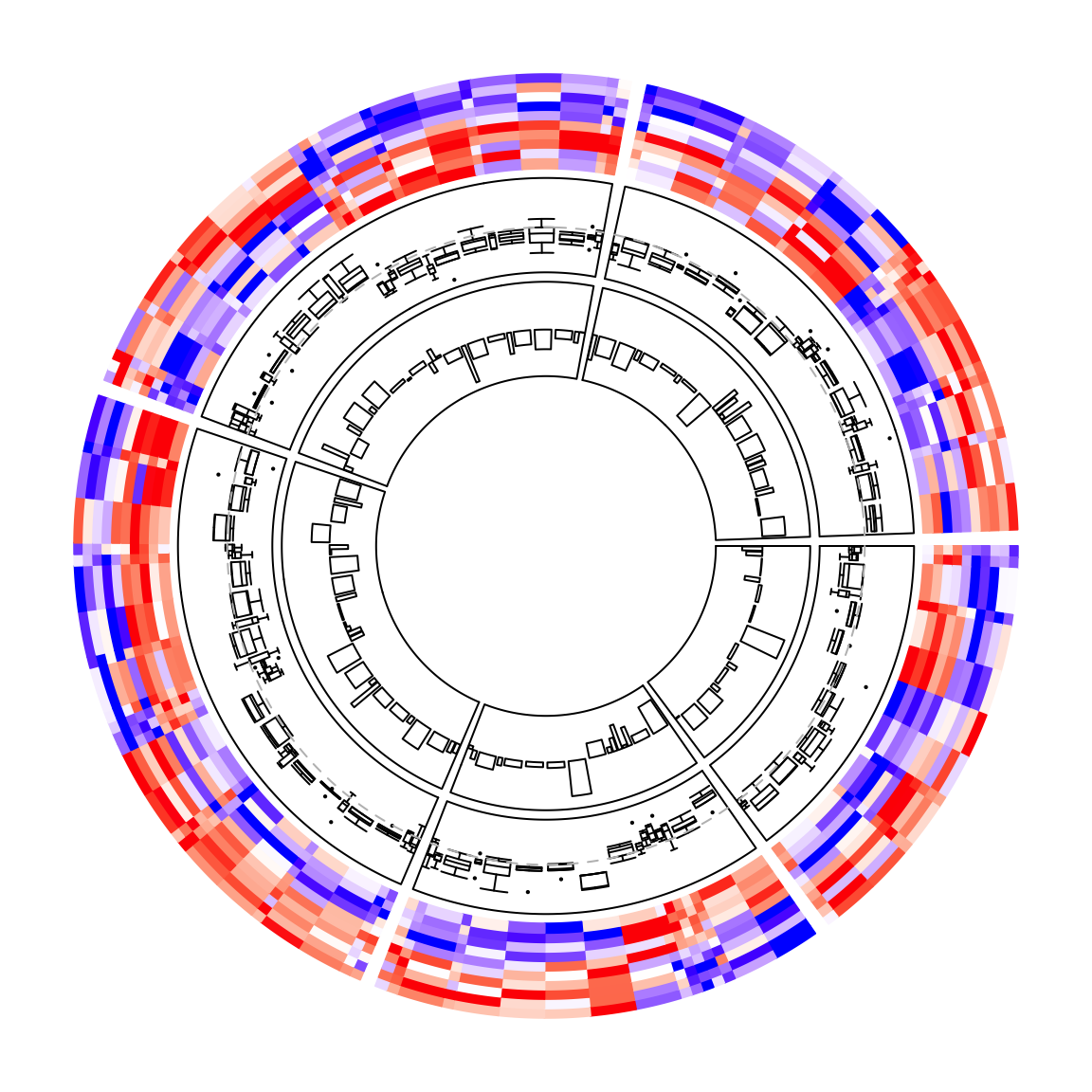circos.clear()

## Session info

sessionInfo()
## R version 4.0.2 (2020-06-22)
## Platform: x86_64-apple-darwin17.0 (64-bit)
## Running under: macOS Catalina 10.15.5
##
## Matrix products: default
## BLAS:   /Library/Frameworks/R.framework/Versions/4.0/Resources/lib/libRblas.dylib
## LAPACK: /Library/Frameworks/R.framework/Versions/4.0/Resources/lib/libRlapack.dylib
##
## locale:
##  en_GB.UTF-8/en_GB.UTF-8/en_GB.UTF-8/C/en_GB.UTF-8/en_GB.UTF-8
##
## attached base packages:
##  stats     graphics  grDevices utils     datasets  methods   base
##
## other attached packages:
##  circlize_0.4.12.1007 GetoptLong_1.0.5     knitr_1.30
##
## loaded via a namespace (and not attached):
##   cluster_2.1.0        magrittr_2.0.1       BiocGenerics_0.34.0
##   IRanges_2.22.2       clue_0.3-57          colorspace_2.0-0
##   rjson_0.2.20         rlang_0.4.8          stringr_1.4.0
##  tools_4.0.2          parallel_4.0.2       grid_4.0.2
##  xfun_0.19            png_0.1-7            htmltools_0.5.0
##  matrixStats_0.57.0   yaml_2.2.1           digest_0.6.27
##  crayon_1.3.4         bookdown_0.21        RColorBrewer_1.1-2
##  S4Vectors_0.26.1     GlobalOptions_0.1.2  shape_1.4.5
##  evaluate_0.14        rmarkdown_2.5        blogdown_0.17
##  ComplexHeatmap_2.7.4 stringi_1.5.3        compiler_4.0.2
##  stats4_4.0.2         Cairo_1.5-12.2